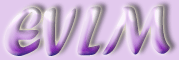Introduction to the EVLM Student Book

The EVLM student book is composed of 25 chapters containing information which should be of value to any students who have some mathematical content in their undergraduate studies. Most of the chapters combine the explanation of theory with the solution of problems using mathematical or statistical packages such as Maple or SPSS. Chapters are arranged in groups of 3 or 4 themed generally themed around one of the packages.

Chapter 2 gives the theory underlying the least-squares method for approximation of data, which is used in regression analysis. Chapter 3 provides the reader with a brief introduction to the statistical analysis package SPSS with Chapters 4 and 5 giving more in-depth understanding of SPSS in the context of cluster analysis.

Chapters 9 - 11 investigate the use of the computer algebra package Mathematica to find solutions for odinary differential equations (ODEs). Chapter 9 employs one-step numerical methods and Chapter 10 uses Runge-Kutta techniques, while Chapter 11 looks at the stability of critical points in linear systems of ODEs.

Chapter 12 provides some tips for students who are specialising in mathematics. Chapter 13 offers an introduction to financial mathematics, introducing such concepts as compound interest and net present value. The chapter contains several worked exercises.

Chapters 14 - 16 are essentially worksheets for those students studying integration techniques. Integration by parts and integration by substitution are explained and several examples are given. Solutions are provided both in long hand form and also in the form of answers using the computer algebra system Maple.

Chapters 17 - 21 again utilise Maple. Chapter 17 provides a brief introduction to the basics of Maple. Chapters 18 and 19 demonstrate respectively the use of Maple in finding derivatives and critical points of functions in one variable and the solution of ODEs.Chapter 20 explains how to work with matrices in Maple and chapter 21 demonstrates usage of Maple for plotting graphs.

Chapters 22 - 24 demonstrate the use of the publishing software Publicon, which has been specifically developed to allow the insertion of active text into technical documents. An explanation of Publicon's capabilities is given in Chapter 22, followed with a start-up guide in Chapter 22. Chapter 23 provides examples of works created by students working with Publicon.

Chapters 25 offers the student a start up guide to the numerical computation package MATLAB. This is followed by Chapter 26, which explains the significance and writing of m-files in MATLAB, while Chapter 27 provides a guide to the annotation of graphs in MATLAB.